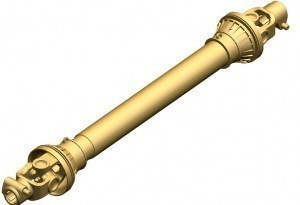# Drive Shaft Dimensions

Whether it is for motorbikes, cars or locomotives,the drive shaft’s functions remain the same: they are utilized for transmitting torque. Examples of drive shaft specs are outlined
below.

### Drive Shaft Dimensions: SPL-55 and SPL-55XS

The minimum length (from centerline cross to end stroke; A) for SPL-55 is 160 mm or 6.28 in. The maximum angle (B) is 21.5 degrees. The tube size is 89 mm or 3.50”. The maximum C angle is 25°. The U-joint span (F) is 106 mm or 4.19 niches. The bearing cup diameter (G) is 35 mm or 1.37 inches.

The SPL-55XS length (centerline cross to the end yoke; A) is 160 mm (equal to 6.28 inches.) The max angle (B) is 21.5 degrees. The tube size is 89 mm or 3.50 inches. The maximum C angle is 25. The U-joint span (F) is 106 mm or 4.19 inches. The bearing cup diameter (G) is 35 mm or 1.37 inches.

### Drive Shaft Dimensions: SPL-70 and SPL-100

The SPL-70 cross centerline length to end yoke (A) is 168 mm or 6.62 inches. The maximum angle (B) is 26 degrees and the tube size is 89 mm or 3.50 or inches. The maximum angle (C) is 25 degrees. The U-joint span (F) is 126 mm or 4.96 inches. The bearing cup diameter (G) is 35 mm (equal to 1.37 inches).

The SPL-100’s minimum length cross centerline to end yoke is 203 mm or 8.00 inches. The maximum B angle is 25. The tube size is 102 mm (equivalent to 4.00 inches). The maximum angle (C) is 15 degrees. The U-joint span is 126 mm (4.96 inches). The bearing cup diameter (G) is 41 mm or 1.63 inches.

### Drive Shaft Dimensions: SPL 250 and SPL-170 I/A

The SPL 250 series has a maximum momentary joint angle of 25 degrees. The standard slip of D/S is 110 mm or 4.33 in. The rotating diameter of D/S is 185 mm or 7.28 in. The rotating diameter of the end yoke is 193 mm or 7.60 in.

The specs for the SPL-170 I/A are the following: the maximum momentary joint angle is 45 degrees. The standard slip of D/S is 150 mm or 5.90 in. The rotating diameter of D/S is 185 mm or 7.28 in. The rotating diameter of end yoke is 193 mm (equal to 7.60 in.)

### Drive Shaft Dimensions: SPL 140 and SPL 170

The SPL 140 has a maximum momentary joint angle of 25 degrees. The standard slip of D/S is 110 mm or 4.33 in. The rotating diameter of D/S is 160 mm (equal to 6.30 in.). The rotating diameter of the end yoke is 158 mm or 6.22 in.

The SPL 170 has the same max momentary joint angle and D/S standard slip. The rotating number of D/S, however, is 185 mm or 7.28 in. The rotating diameter of the end yoke is 193 mm or 7.60 in.

How much you know about drive shaft dimensions will go a long way towards helping you maintain your vehicle. That being said, it’s still a good idea to have the car checked every now and then to avoid problems.